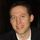cancel
Showing results for
Did you mean:Frequent Visitor

## Alternative LookupValue function to create virtual relationship.

Developing my first power bi data model. Below tables are part of my data model.

 FxRatePeriod Currency Rate Jan-22 Euro 1.05 Jan-22 GBP 1.2 Feb-22 Euro 1.03 Feb-22 GBP 1.1

 TrxID Currency OrigAmount 001 Euro 75 002 Euro 85 003 GBP 78 004 GBP 34

Measures: convertedAmount = OrigAmount*Rate

PrevMonthRateConvertedAmount = OrigAmount*PrevMonthRatePeriod

I need measure that can be dynamically calculated based on FxRate period selected by users.

I can make it work using LookupValue and wanted to know if there is better way doing it perhaps TREATAS as performances might become an issue.

Any suggestion greatly appreciated.

1 ACCEPTED SOLUTIONSuper User

This solution uses a Currency table that functions as a bridge table between the ExchangeRate and Transaction tables. The Currency table can be created in DAX or Power Query. The column ExchangeRate[FxRatePeriod] uses the first day of the month.Measures:

``````ConvertedAmount =
SUMX (
'Transaction',
VAR vRate =
MAXX ( RELATEDTABLE ( ExchangeRate ), ExchangeRate[Rate] )
RETURN
'Transaction'[OrigAmount] * vRate
)``````
``````PrevMonthRateConvertedAmount =
SUMX (
'Transaction',
VAR vCurrency = 'Transaction'[Currency]
VAR vPeriod =
EDATE ( SELECTEDVALUE ( ExchangeRate[FxRatePeriod] ), -1 )
VAR vTable =
FILTER (
ALL ( ExchangeRate ),
ExchangeRate[Currency] = vCurrency
&& ExchangeRate[FxRatePeriod] = vPeriod
)
VAR vRate =
MAXX ( vTable, ExchangeRate[Rate] )
RETURN
'Transaction'[OrigAmount] * vRate
)``````

Visual:Proud to be a Super User!Super User

This solution uses a Currency table that functions as a bridge table between the ExchangeRate and Transaction tables. The Currency table can be created in DAX or Power Query. The column ExchangeRate[FxRatePeriod] uses the first day of the month.Measures:

``````ConvertedAmount =
SUMX (
'Transaction',
VAR vRate =
MAXX ( RELATEDTABLE ( ExchangeRate ), ExchangeRate[Rate] )
RETURN
'Transaction'[OrigAmount] * vRate
)``````
``````PrevMonthRateConvertedAmount =
SUMX (
'Transaction',
VAR vCurrency = 'Transaction'[Currency]
VAR vPeriod =
EDATE ( SELECTEDVALUE ( ExchangeRate[FxRatePeriod] ), -1 )
VAR vTable =
FILTER (
ALL ( ExchangeRate ),
ExchangeRate[Currency] = vCurrency
&& ExchangeRate[FxRatePeriod] = vPeriod
)
VAR vRate =
MAXX ( vTable, ExchangeRate[Rate] )
RETURN
'Transaction'[OrigAmount] * vRate
)``````

Visual:Proud to be a Super User!